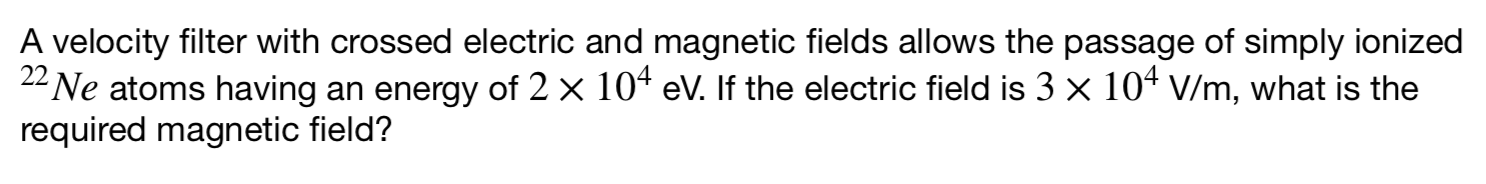Home / Expert Answers / Physics / a-velocity-filter-with-crossed-electric-and-magnetic-fields-allows-the-passage-of-simply-ionized-pa470

# (Solved): A velocity filter with crossed electric and magnetic fields allows the passage of simply ionized \ ...A velocity filter with crossed electric and magnetic fields allows the passage of simply ionized $${ }^{22} \mathrm{Ne}$$ atoms having an energy of $$2 \times 10^{4} \mathrm{eV}$$. If the electric field is $$3 \times 10^{4} \mathrm{~V} / \mathrm{m}$$, what is the required magnetic field?

We have an Answer from Expert